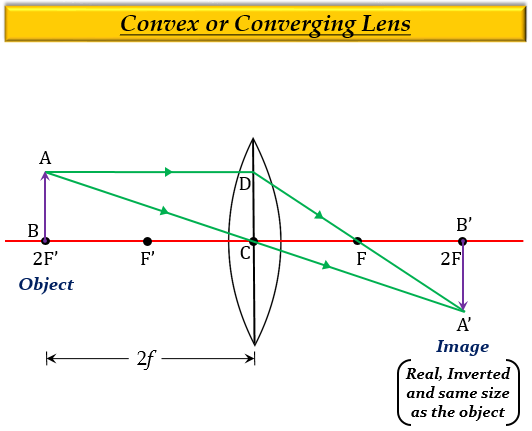# A burning candle whose flame is 1.5 cm tall is placed at a certain distance in front of a convex lens. An image of candle flame is received on a white screen kept behind the lens. The image of flame also measures 1.5 cm. If f is the focal length of convex lens, the candle is placed:(a) at f (b) between f and 2f (c) at 2f (d) beyond 2f

(c) at 2f

Explanation

When an object is at $(2F')$ of a convex lens, which means the object is at a distance equal to twice the focal length, $2f$ of a convex lens, the image formed is real, inverted, same size as the object, and at a distance $2f$ on the other side of the lens.